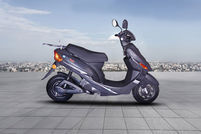# Avon E Mate EMI CalculatorRs. 45,000*
*Ex-showroom Price in delhi

Avon E Mate EMI starts at Rs 1,583 per month for a tenure of 36 months @9.7 for a loan amount of Rs 44,219. The EMI Calculator tool on BikeDekho gives a detailed break-up of the total payable amount and helps you in finding the best bike finance for your E Mate. (Calculated on On Road Price in Delhi)

## Avon E Mate Down Payment and EMI

E Mate VariantsLoan @ 9.7%. Down paymentEMI (36 months)
STD44,219Rs. 4,913Rs. 1,583

## Calculate your Loan EMI for E Mate

Down PaymentRs.
0Rs.0
Bank Interest Rate %
8%26%
Loan Period ( Months )
• Total Loan AmountRs.0
• Payable AmountRs.0
• You’ll pay extraRs.0
EMIper month
Rs0
Calculated on On Road Price in delhi

## Find EMI of E Mate Alternatives

*Ex-showroom Price in delhi

## E Mate EMI FAQs

• FAQ

### What will be the lowest down payment for Avon E Mate?

The lenders generally finance 90% of the value of the . Some customers might be eligible for 100% funding too. The down payment is the difference between the on-road price of the and the amount funded by a lender. This means the minimum possible down payment that you have to pay for Avon E Mate includes the RTO and insurance charges.

### What will be the monthly EMI for Avon E Mate?

EMIs or Equated Monthly Installments refer to the monthly payments you make to the lender to repay your loan. These payments include the principal amount as well as the interest i.e. EMI = Principal Amount + Interest on Principal amount. Mathematically, EMI for Avon E Mate can be calculated using the following formula:

{P x R x (1+R)^N / [(1+R)^N-1]}

where, P = Principal amount of the loan, R = Rate of interest and N = Number of monthly installments.

For Example:- If the principal amount for a bike loan is Rs. ₹44,132/- on an annual rate of interest of 10% for a tenure of 3 years then

EMI = 44132 * 0.008333*(1+ 0.008333)^36 / ((1+ 0.008333)^36)-1 = Rs /-

The rate of interest (R) on your loan is calculated monthly i.e. (R= Annual rate of interest/12/100). For instance, if R = 10% per annum, then R= 10%/12 = 0.008333.

### What will be the interest rate for Avon E Mate?

The interest rate for Avon E Mate primarily depends on the principal amount and tenure of the loan amount. Interest rate of lenders generally varies from 9.7% per annum to 15% per annum. Customers can also negotiate with the financier for a better rate of interest against their loan amount.

### What will be the EMI for 3 years tenure for Avon E Mate?

EMI for Avon E Mate for 3 years tenure can be calculated as per the following formula:-

{P x R x (1+R)^N / [(1+R)^N-1]}

Where, P = Principal amount of the loan, R = Rate of interest, N = Number of monthly installments which will be 36 in case of 3 years tenure.

## Top 10 Scooters in India

•TVS Jupiter
Rs64,437 - 73,737*
•Honda Activa 6G
Rs67,843 - 71,089*
•Honda Dio
Rs63,273 - 69,171*
•Suzuki Access 125
Rs71,000 - 80,200*
•TVS NTORQ 125
Rs71,095 - 81,075*
•TVS XL100
Rs41,015 - 50,384*
• ElectricAther 450X
Rs1.27 - 1.46 Lakh*
•Hero Pleasure Plus
Rs58,900 - 64,100*
• ElectricBajaj Chetak
Rs1 - 1.15 Lakh*
•Yamaha Fascino 125
Rs72,030 - 75,530*
*Ex-showroom Price in delhi

## Trending Avon Scooters

*Ex-showroom price in Delhi

## E Mate Running Cost

KM driven per day20 km/day
Monthly fuel costRs.0* / month
Disclaimer :

Disclaimer : As per the information entered by you the calculation is performed by EMI Calculator and the amount of installments does not includes any other fees charged by the financial institution / banks like processing fee, file charges, etc. The amount is in Indian Rupee rounded off to the nearest Rupee. Depending upon type and use of vehicle, regional lender requirements and the strength of your credit, actual down payment and resulting monthly payments may vary. Exact monthly installments can be found out from the financial institution.